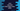# Java Program to calculate BMI or Body Mass Index## Java program to calculate BMI ( Body Mass Index ):

BMI or body mass index can be calculated by taking the weight in pounds and height in inches or by taking the weight in kilograms and height in meters. We have to use a different formula for both cases. In this example, we will learn how to do the calculation by using any of these two different approaches.

### BMI using weight in pound and height in inches :

The BMI for pound/inches is :

``````BMI = Weight(pound) * 730 /height (inches) * height (inches)
``````

### BMI using weight in Kilogram and height in Meters :

The BMI for Kilogram/Meters is :

``````BMI = Weight(Kilogram)/height (Meters) * height (Meters)
``````

We will also print if the result falls under ‘underweight’, ‘normal’, ‘overweight’ or ‘obese’.

For that, let’s first calculate the BMI value and then check on which range it falls :

``````Less than 18.5 = Underweight
Between 18.5 to 24.9 = Normal
Between 25 to 29.9 = Overweight
30 or more than 30 = Obese``````
• In the program below, we have two different methods to calculate the BMI by using the above methods.
• For weight/height in pounds/inches , ’calculateBMImethodOne’ will be called and for weight/height in kilogram/meters , ’calculateBMImethodTwo’ will be called.
• We will ask the user either he wants to use approach 1 or 2. If he enters 1, call the first method and for 2, call method 2.
• Finally, pass the calculated BMI value to ’printBMIResult’ method and print out a formatted string about the result.
• You will get more clarification after going through the program :

### Java Program :

``````package com.company;

import java.util.Scanner;

public class Main {

/**
* Utility functions
*/
static void println(String string) {
System.out.println(string);
}

static void print(String string) {
System.out.print(string);
}

/**
* Print the final result
* @param bmi : calculated BMI result
*/
private static void printBMIResult(float bmi) {
String result = "";

if (bmi < 18.5) {
result = "underweight";
} else if (bmi < 25) {
result = "normal";
} else if (bmi < 30) {
result = "overweight";
} else {
result = "obese";
}

println("Your BMI is " + bmi + " and that means " + result);

}

/**
* calculate BMI if weight/height in pounds/inches
*/
private static void calculateBMImethodOne() {
Scanner scanner = new Scanner(System.in);
println("Enter weight in Pounds : ");
float weight = scanner.nextFloat();

println("Enter Height in inches : ");
float height = scanner.nextFloat();

float bmi = (weight * 703) / (height * height);

//print the result
printBMIResult(bmi);

}

/**
* calculate BMI if weight/height in kilogram/meters
*/
private static void calculateBMImethodTwo() {
Scanner scanner = new Scanner(System.in);
println("Enter weight in Kilogram : ");
float weight = scanner.nextFloat();

println("Enter Height in Meters : ");
float height = scanner.nextFloat();

float bmi = (weight) / (height * height);

//print the result
printBMIResult(bmi);
}

public static void main(String[] args) {
Scanner sc = new Scanner(System.in);

println("You can calculate BMI by entering weight in pounds and height in inches or Weight in Kilograms and Height in Meter.");

while (true) {
println("");
println("Enter 1 for entering weight/height in pounds/inches OR 2 for kilogram/meters : ");
int userInput;

userInput = sc.nextInt();

if (userInput == 1) {
calculateBMImethodOne();
break;
} else if (userInput == 2) {
calculateBMImethodTwo();
break;
} else {
println("Invalid Input !!!");
continue;
}
}
}
}``````

### Sample Example Output :

``````You can calculate BMI by entering weight in pounds and height in inches or Weight in Kilograms and Height in Meter.

Enter 1 for entering weight/height in pounds/inches OR 2 for kilogram/meters :
1
Enter weight in Pounds :
1234
Enter Height in inches :
23
Your BMI is 1639.8904 and that means obese

Enter 1 for entering weight/height in pounds/inches OR 2 for kilogram/meters :
2
Enter weight in Kilogram :
40
Enter Height in Meters :
6
Your BMI is 1.1111112 and that means underweight

Enter 1 for entering weight/height in pounds/inches OR 2 for kilogram/meters :
2
Enter weight in Kilogram :
60
Enter Height in Meters :
1.7
Your BMI is 20.761246 and that means normal
``````